Integers 1
(10 Questions)
CA Math Standards
Grade 5
2.1 Add, subtract, multiply and divide with decimals; add with negative numbers; subtract positive integers from negative integers; verify the reasonableness of the results.
From Mr. Anker Tests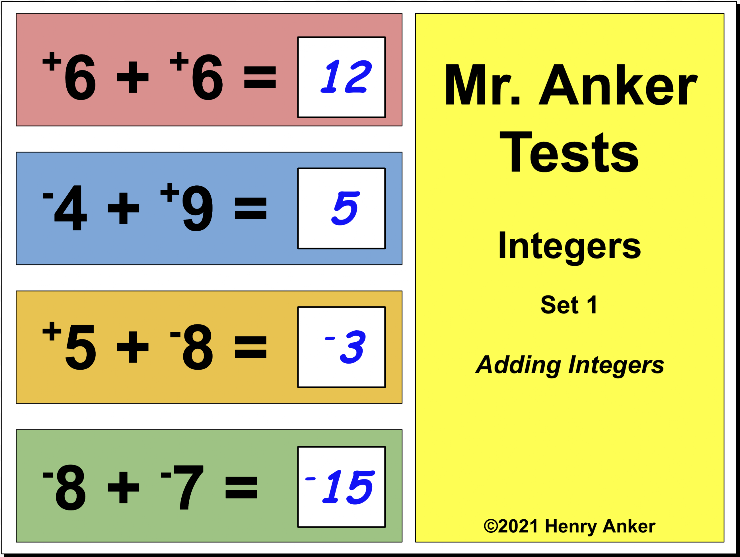Integer Number Line Reference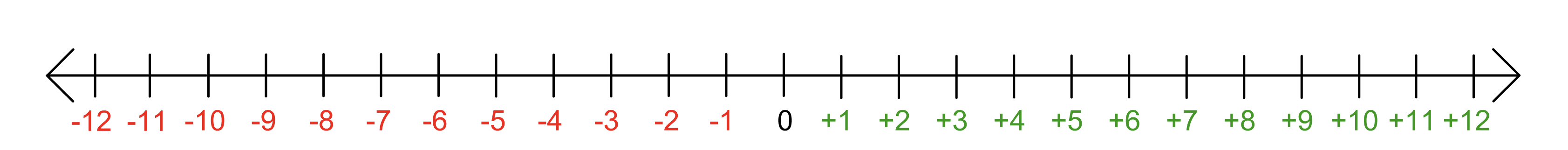*Note
Small positive signs (+) will appear next to positive integers in this first set only, to help assure that the student is aware that the number associated with the sign is positive.
1. Which of the choices below is the correct solution to this equation? *
1 point2. In the space provided below, type the correct solution to this equation. *
1 point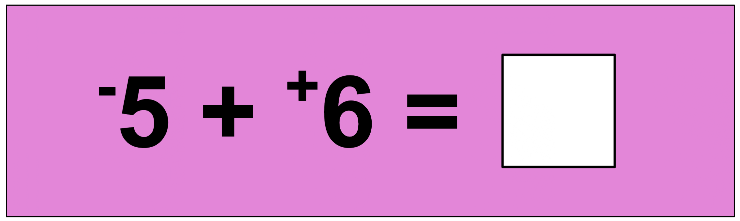*Use the minus/subtraction sign with negative integer answers, like this... -10
3. Which of the choices below is the correct solution to this equation? *
1 point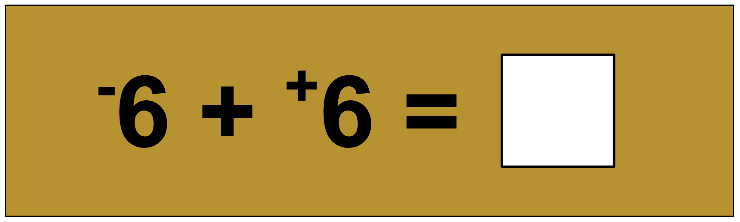4. In the space provided below, type the correct solution to this equation. *
1 point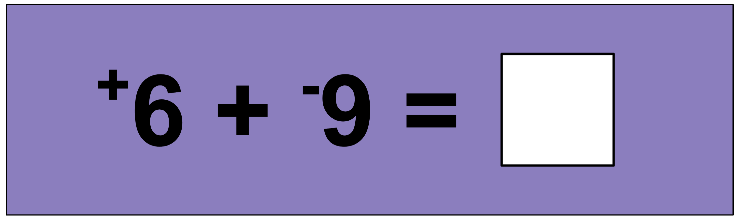*Use the minus/subtraction sign with negative integer answers, like this... -10
5. Which of the choices below is the correct solution to this equation? *
1 point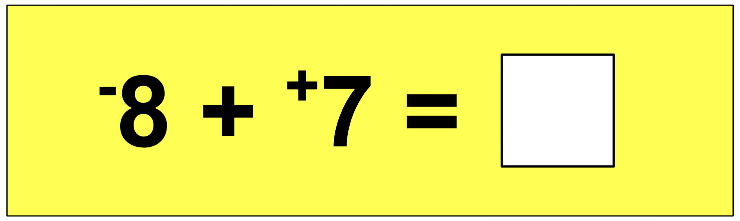6. In the space provided below, type the correct solution to this equation. *
1 point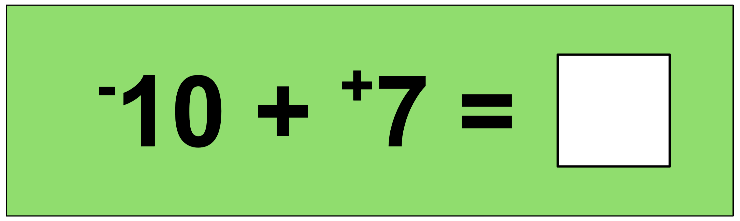7. Which of the choices below is the correct solution to this equation? *
1 point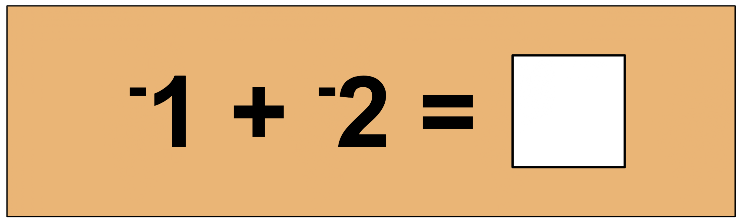8. In the space provided below, type the correct solution to this equation. *
1 point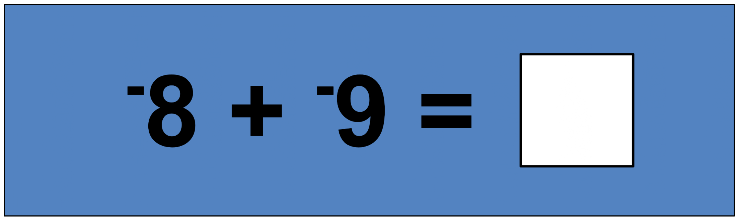9. Which choice makes the number sentence true? *
1 point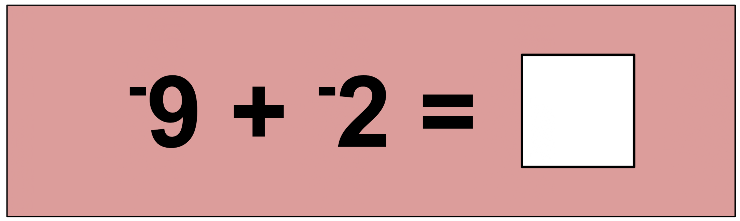10. In the space provided below, type the correct solution to this equation. *
1 point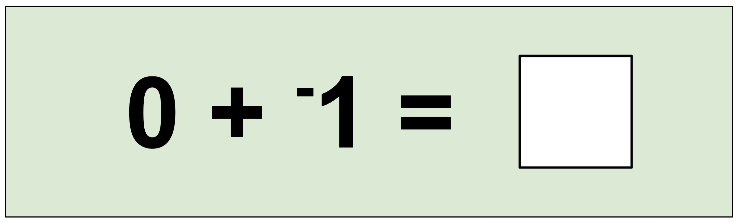Never submit passwords through Google Forms.
This content is neither created nor endorsed by Google. Report Abuse - Terms of Service - Privacy Policy